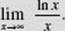﻿ ﻿INDETERMINATE FORMS AND L’HÔPITAL’S RULE - Differentiation - Calculus AB and Calculus BC

## CHAPTER 3 Differentiation

### J.* INDETERMINATE FORMS AND L’HÔPITAL’S RULE

BC ONLY

Limits of the following forms are called indeterminate:To find the limit of an indeterminate form of the typewe apply L’Hôpital’s Rule, which involves taking derivatives of the functions in the numerator and denominator. In the following, a is a finite number. The rule has several parts:

(a) Ifand ifexists, thenifdoes not exist, then L’Hôpital’s Rule cannot be applied.

* Although this a required topic only for BC students, AB students will find L’Hôpital’s Rule very helpful.

The limit can be finite or infinite (+∞ or −∞).

(b) Ifthe same consequences follow as in case (a). The rules in (a) and (b) both hold for one-sided limits.

(c) Ifexists, thenifdoes not exist, then L’Hôpital’s Rule cannot be applied. (Here the notation “x → ∞” represents either “x → + ∞” or “x → −∞.”)

(d) Ifthe same consequences follow as in case (c).

In applying any of the above rules, if we obtainagain, we can apply the rule once more, repeating the process until the form we obtain is no longer indeterminate.

Examples 38–43 are BC ONLY.

EXAMPLE 38is of typeand thus equals(Compare with Example 12 from Chapter 1.)

EXAMPLE 39is of typeand therefore equalsEXAMPLE 40(Example 13) is of typeand thus equalsas before. Note thatis not the limit of an indeterminate form!

EXAMPLE 41is of typeand therefore equalsEXAMPLE 42(Example 20) is of typeso that it equalswhich is again of typeApply L’Hôpital’s Rule twice more:For this problem, it is easier and faster to apply the Rational Function Theorem!

EXAMPLE 43

FindSOLUTION:is of typeand equalsEXAMPLE 44

FindSOLUTION:BEWARE: L’Hôpital’s Rule applies only to indeterminate formsTrying to use it in other situations leads to incorrect results, like this:L’Hôpital’s Rule can be applied also to indeterminate forms of the types 0 · ∞ and ∞ − ∞, if the forms can be transformed to eitherEXAMPLE 45

FindSOLUTION:is of the type ∞ · 0. Since xand, as x → ∞, the latter is the indeterminate formwe see that(Note the easier solutionOther indeterminate forms, such as 00, 1 and ∞0, may be resolved by taking the natural logarithm and then applying L’Hôpital’s Rule.

BC ONLY

EXAMPLE 46

FindSOLUTION:is of type 1. Let y = (1 + x)1/x, so that

lnln (1 + x). Thenlnwhich is of typeThus,and sinceln y = 1,y = e1 = e.

EXAMPLE 47

FindSOLUTION:is of type ∞0. Let y = x1/x, so that ln(which, as x → ∞, is of type). Thenlnandy = e0 = 1.

For more practice, redo the Practice Exercises, applying L’Hôpital’s Rule wherever possible.

﻿